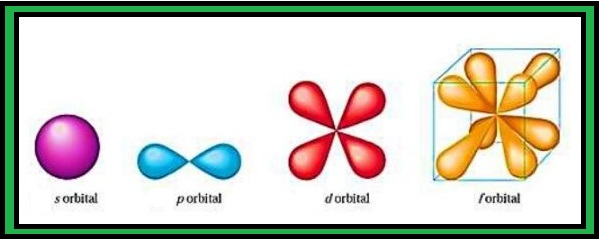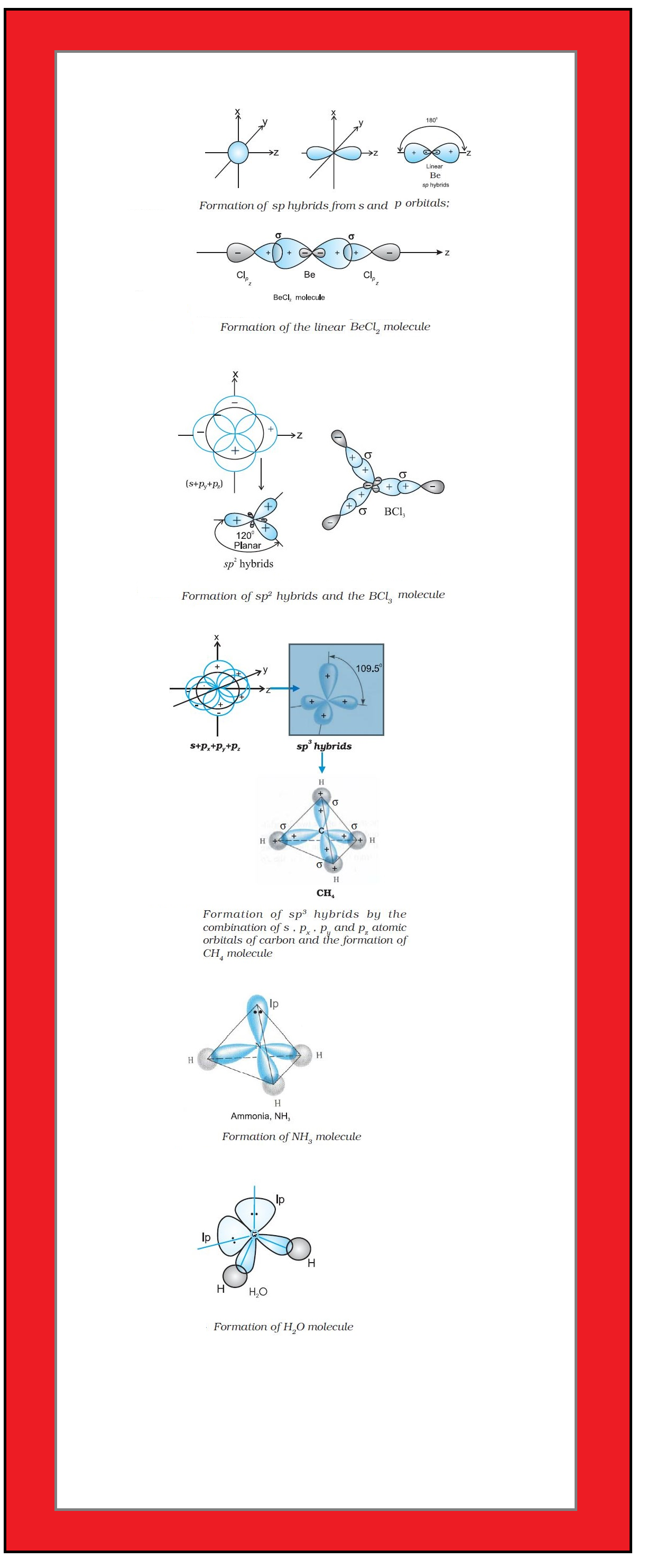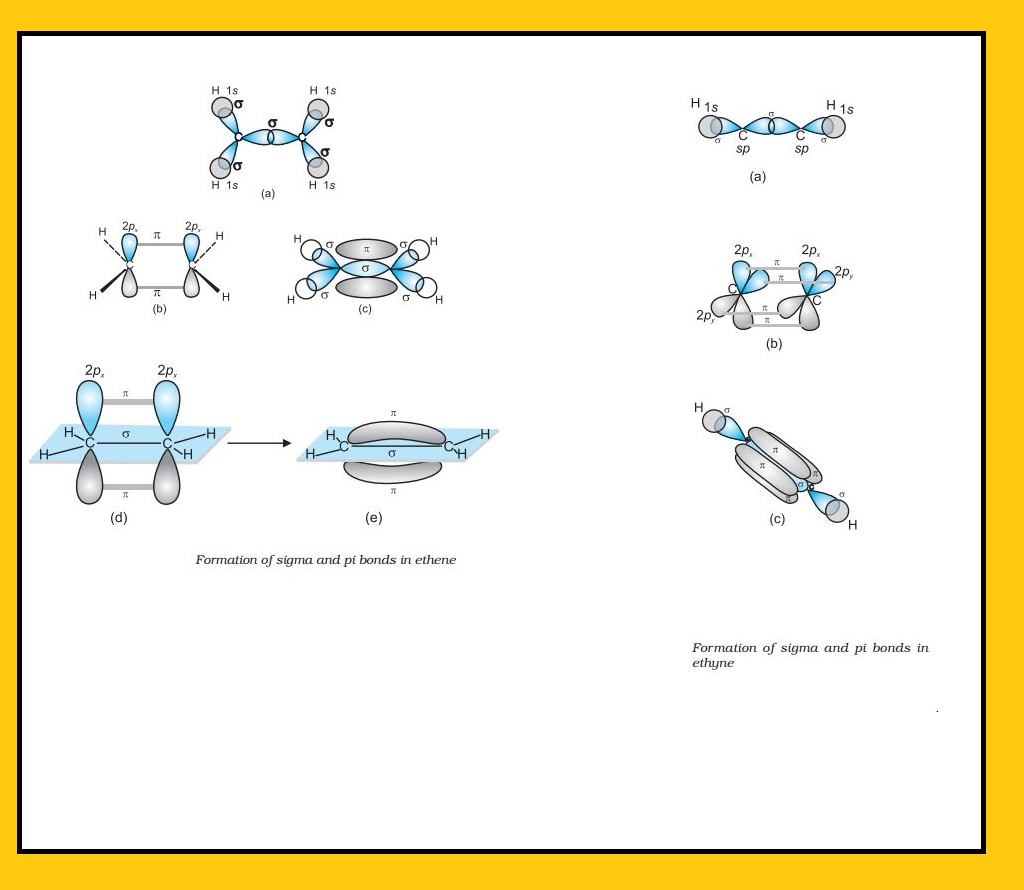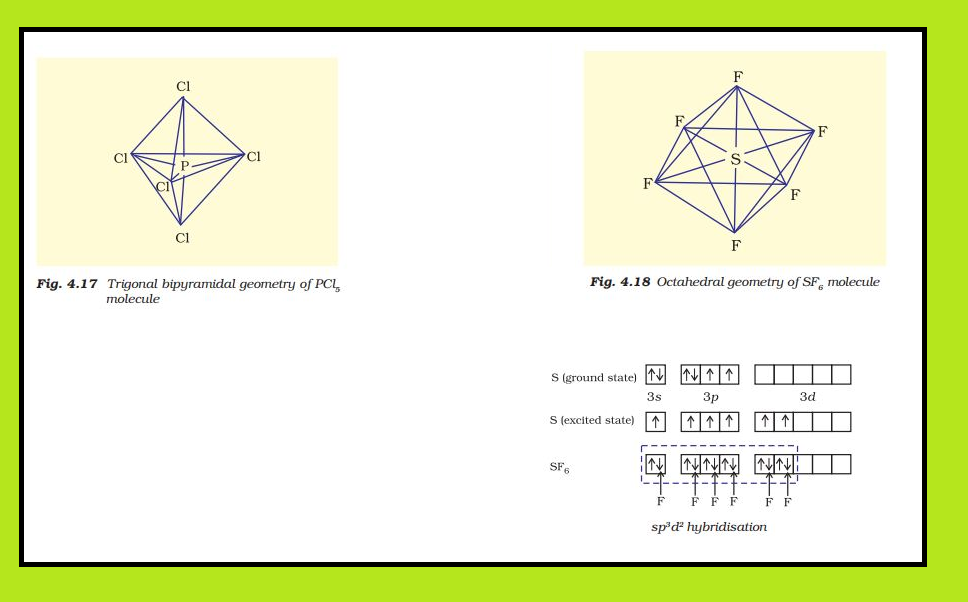Chemistry Hybridisation
Click for Only Video

### Topics Covered :● Hybridiasation
● Types of Hybridisation
● Other Examples of sp^3, sp^2 and sp Hybridisation
● Hybridisation of Elements Involoving d-orbitals

### Hybridisation :

=> In order to explain the characteristic geometrical shapes of polyatomic molecules like CH_4, NH_3 and H_2O etc., Pauling introduced the concept of hybridisation.

=> According to him, the atomic orbitals combine to form new set of equivalent orbitals known as text(hybrid orbitals).

● Unlike pure orbitals, the hybrid orbitals are used in bond formation.

color{purple}♣ color{Violet} " Just for Curious"
If we mix one can of white paint with one can of red paint, we get two cans of pink paint which are exactly identical. Pink colour is an intermediate of white and red colours and hence can be considered as a hybrid of red and white.

color{purple}(✓✓)color{purple} " DEFINITION ALERT"

color{green}("Hybridisation ") It can be defined as the process of intermixing of the orbitals of slightly different energies so as to redistribute their energies, resulting in the formation of new set of orbitals of equivalent energies and shape.

color{red}("Example" ) When one 2s and three 2p-orbitals of carbon hybridise, there is the formation of four new sp^3 hybrid orbitals.

### Salient features of hybridisation :

The main features of hybridisation are as under :

=> Only those orbitals which have approximately equal energies and belong to the same atom can undergo hybridisation.

=> Number of hybrid orbitals produced is equal to the number of atomic orbitals mixed.

=> The number of hybrid orbitals is equal to the number of the atomic orbitals that get hybridised.

=> Hybridisation never takes place in isolated atoms but it occurs only at the time of bond formation.

=> The hybridised orbitals are always equivalent in energy and shape.

=> The hybrid orbitals are more effective in forming stable bonds than the pure atomic orbitals.

=> These hybrid orbitals are directed in space in some preferred direction to have minimum repulsion between electron pairs and thus a stable arrangement. The type of hybridisation indicates the geometry of the molecules.

### Important conditions for hybridisation :

(i) The orbitals present in the valence shell of the atom are hybridised.

(ii) The orbitals undergoing hybridisation should have almost equal energy.

(iii) Promotion of electron is not essential condition prior to hybridisation.

(iv) It is not necessary that only half filled orbitals participate in hybridisation. In some cases, even filled orbitals of valence shell take part in hybridisation.

### Types of Hybridisation :There are various types of hybridisation involving s, p and d orbitals. The different types of hybridisation are as under :

(i) color{green}("sp Hybridisation ") This type of hybridisation involves the mixing of one s and one p orbital resulting in the formation of two equivalent sp hybrid orbitals.

=> The suitable orbitals for sp hybridisation are s and p_z, if the hybrid orbitals are to lie along the z-axis.

=> Each sp hybrid orbitals has 50% s-character and 50% p-character.

=> Molecule in which the central atom is sp-hybridised and linked directly to two other central atoms possesses linear geometry.

=> This type of hybridisation is also known as diagonal hybridisation.

=> The two sp hybrids point in the opposite direction along the z-axis with projecting positive lobes and very small negative lobes, which provides more effective overlapping resulting in the formation of stronger bonds.

color{red}("Example ") BeCl_2

● The ground state electronic configuration of Be is 1s^2 2s^2.

● In the exited state one of the 2s-electrons is promoted to vacant 2p orbital to account for its divalency.

● One 2s and one 2p-orbitals get hybridised to form two sp hybridised orbitals.

● These two sp hybrid orbitals are oriented in opposite direction forming an angle of 180°.

● Each of the sp hybridised orbital overlaps with the 2p-orbital of chlorine axially and form two Be- Cl sigma bonds. This is shown in Fig. 4.10.

(ii) color{green}( sp^2 "Hybridisation ") In this hybridisation there is involvement of one s and two p-orbitals in order to form three equivalent sp^2 hybridised orbitals.

color{red}("Example ") BCl_3 molecule

● The ground state electronic configuration of central boron atom is 1s^2 2s^2 2p^1.

● In the excited state, one of the 2s electrons is promoted to vacant 2p orbital as a result boron has three unpaired electrons.

● These three orbitals (one 2s and two 2p) hybridise to form three sp^2 hybrid orbitals.

● The three hybrid orbitals so formed are oriented in a trigonal planar arrangement and overlap with 2p orbitals of chlorine to form three B-Cl bonds.

● Therefore, in BCl_3 (Fig. 4.11), the geometry is trigonal planar with Cl B Cl bond angle of 120°.

(iii) color{green}(sp^3 ("Hybridisation ") This type of hybridisation can be explained by taking the example of CH_4 molecule in which there is mixing of one s-orbital and three p-orbitals of the valence shell to form four sp^3 hybrid orbital of equivalent energies and shape.

● There is 25% s-character and 75% p-character in each sp^3 hybrid orbital.

● The four sp^3 hybrid orbitals so formed are directed towards the four corners of the tetrahedron.

● The angle between sp^3 hybrid orbital is 109.5° as shown in Fig. 4.12.

=> The structure of NH_3 and H_2O molecules can also be explained with the help of sp^3 hybridisation.

● In NH_3, the valence shell (outer) electronic configuration of nitrogen in the ground state is 2s^2 2 p_x^1 2 p_y^1 2p_z^1 having three unpaired electrons in the sp^3 hybrid orbitals and a lone pair of electrons is present in the fourth one.

● These three hybrid orbitals overlap with 1s orbitals of hydrogen atoms to form three N–H sigma bonds. We know that the force of repulsion between a lone pair and a bond pair is more than the force of repulsion between two bond pairs of electrons.

● The molecule thus gets distorted and the bond angle is reduced to 107° from 109.5°.

● The geometry of such a molecule will be pyramidal as shown in Fig. 4.13.

=> In case of H_2O molecule, the four oxygen orbitals (one 2s and three 2p) undergo sp^3 hybridisation forming four sp^3 hybrid orbitals out of which two contain one electron each and the other two contain a pair of electrons.

● These four sp^3 hybrid orbitals acquire a tetrahedral geometry, with two corners occupied by hydrogen atoms while the other two by the lone pairs.

● The bond angle in this case is reduced to 104.5° from 109.5° (Fig. 4.14) and the molecule thus acquires a V-shape or angular geometry.

### Other examples of sp^3 , sp^2 and sp Hybridisation.sp^3 Hybridisation in C_2H_6 molecule : In ethane molecule both the carbon atoms assume sp^3 hybrid state. One of the four sp3 hybrid orbitals of carbon atom overlaps axially with similar orbitals of other atom to form sp^3-sp^3 sigma bond while the other three hybrid orbitals of each carbon atom are used in forming sp^3–s sigma bonds with hydrogen atoms. Therefore in ethane C–C bond length is 154 pm and each C–H bond length is 109 pm.

sp^2 Hybridisation in C_2H_4 : In the formation of ethene molecule, one of the sp^2 hybrid orbitals of carbon atom overlaps axially with sp^2 hybridised orbital of another carbon atom to form C–C sigma bond. While the other two sp^2 hybrid orbitals of each carbon atom are used for making sp^2–s sigma bond with two hydrogen atoms. The unhybridised orbital (2px or 2py) of one carbon atom overlaps sidewise with the similar orbital of the other carbon atom to form weak π bond, which consists of two equal electron clouds distributed above and below the plane of carbon and hydrogen atoms.

Thus, in ethene molecule, the carboncarbon bond consists of one sp^2–sp^2 sigma bond and one pi (π ) bond between p orbitals which are not used in the hybridisation and are perpendicular to the plane of molecule; the bond length 134 pm. The C–H bond is sp^2–s sigma with bond length 108 pm. The H– C–H bond angle is 117.6° while the H–C–C angle is 121°. The formation of sigma and pi bonds in ethene is shown in Fig.

sp Hybridisation in C_2H_2 : In the formation of ethyne molecule, both the carbon atoms undergo sp-hybridisation having two
unhybridised orbital i.e., 2p_y and 2p_x.

One sp hybrid orbital of one carbon atom overlaps axially with sp hybrid orbital of the other carbon atom to form C–C sigma bond, while the other hybridised orbital of each carbon atom overlaps axially with the half filled s orbital of hydrogen atoms forming σ bonds. Each of the two unhybridised p orbitals of both the carbon atoms overlaps sidewise to form two π bonds between the carbon atoms. So the triple bond between the two carbon atoms is made up of one sigma and two pi bonds as shown in Fig.

### Hybridisation of Elements involving d OrbitalsThe elements present in the third period contain d orbitals in addition to s and p orbitals. The energy of the 3d orbitals are comparable to the energy of the 3s and 3p orbitals. The energy of 3d orbitals are also comparable to those of 4s and 4p orbitals. As a consequence the hybridisation involving either 3s, 3p and 3d or 3d, 4s and 4p is possible. However, since the difference in energies of 3p and 4s orbitals is significant, no hybridisation involving 3p, 3d and 4s orbitals is possible.

The important hybridisation schemes involving s, p and d orbitals are summarised
below:

(i) Formation of PCl_5 (sp^3d hybridisation): The ground state and the excited state outer
electronic configurations of phosphorus (Z=15) are represented below.

Now the five orbitals (i.e., one s, three p and one d orbitals) are available for hybridisation to yield a set of five sp3d hybrid orbitals which are directed towards the five corners of a trigonal bipyramidal as depicted in the Fig.

It should be noted that all the bond angles in trigonal bipyramidal geometry are not equivalent. In PCl_5 the five sp^3d orbitals of phosphorus overlap with the singly occupied p orbitals of chlorine atoms to form five P–Cl sigma bonds. Three P–Cl bond lie in one plane and make an angle of 120° with each other; these bonds are termed as equatorial bonds. The remaining two P–Cl bonds–one lying above and the other lying below the equatorial plane, make an angle of 90° with the plane. These bonds are called axial bonds. As the axial bond pairs suffer more repulsive interaction from the equatorial bond pairs, therefore axial bonds have been found to be slightly longer and hence slightly weaker than the equatorial bonds; which makes PCl_5 molecule more reactive.

(ii) Formation of SF_6 (sp^3d^2 hybridisation) : In SF_6 the central sulphur atom has the ground state outer electronic configuration 3s^2 3p^4. In the exited state the available six orbitals i.e., one s, three p and two d are singly occupied by electrons. These orbitals hybridise to form six new sp^3d^2 hybrid orbitals, which are projected towards the six corners of a regular octahedron in SF_6. These six sp^3d^2

hybrid orbitals overlap with singly occupied orbitals of fluorine atoms to form six S–F sigma bonds. Thus SF_6 molecule has a regular octahedral geometry as shown in Fig.